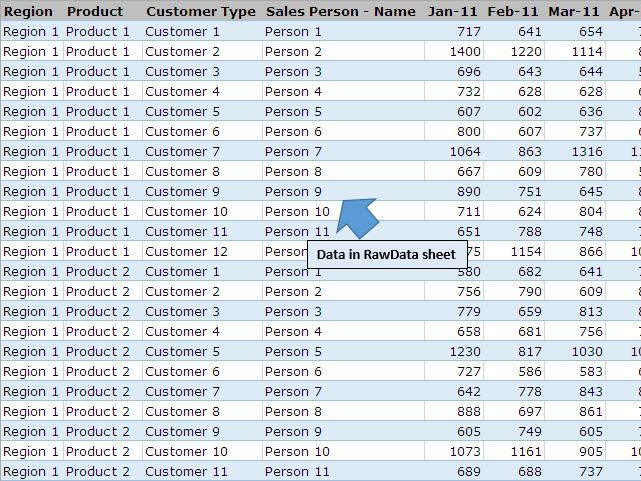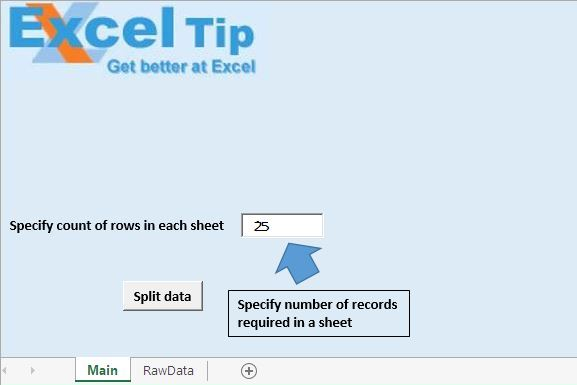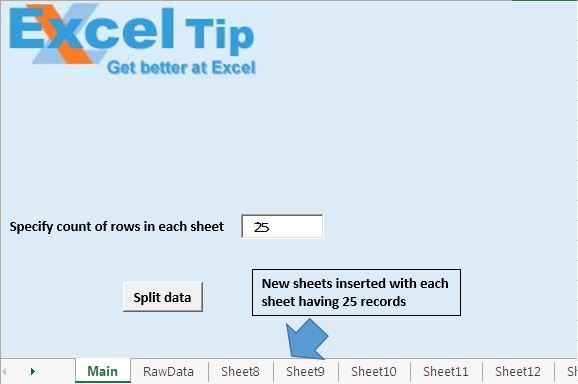# Split data in a sheet by specific number of rows using VBA in Microsoft Excel

In this article, we will create a macro to split data by specific number of rows.

We have raw data in sheet “RawData”. We want to split this data in multiple sheets.Before running the macro, we need to specify the number of rows required in each sheet.Code explanation

CntRows = Cint(Sheets("Main").TextBox1.Value)

The above code is used to get the count of number of sheets required in a sheet.

LastRow = .Range("A" & .Rows.Count).End(xlUp).Row

The above code is used to get the row number of the last cell.

The above code is used to add a new sheet after the last sheet.

.Range("A" & n).Resize(CntRows, LastColumn).Copy Range("A1")

The above code is used to copy specified number of rows to a new worksheet.

```
Option Explicit

Sub SplitDataToMultipleSheets()

'Declaring variables
Dim LastRow As Long, n As Long, CntRows As Long
Dim LastColumn As Integer

'Getting count of number of rows required in one sheet
CntRows = CInt(Sheets("Main").TextBox1.Value)

Application.ScreenUpdating = False

With Sheets("RawData")

'Getting row number and column number of last cell
LastRow = .Range("A" & .Rows.Count).End(xlUp).Row
LastColumn = .Range("A1").SpecialCells(xlCellTypeLastCell).Column

'Looping through data in the sheet
For n = 1 To LastRow Step CntRows

'Copying data to new worksheet
.Range("A" & n).Resize(CntRows, LastColumn).Copy Range("A1")
Next n

.Activate
End With

Application.ScreenUpdating = True

End Sub

```

We would love to hear from you, do let us know how we can improve our work and make it better for you. Write to us at info@exceltip.com

1.